## Memory Organization in Computer Architecture-

Before you go through this article, make sure that you have gone through the previous article on Memory Hierarchy.

In a computer,

• Memory is organized at different levels.
• CPU may try to access different levels of memory in different ways.
• On this basis, the memory organization is broadly divided into two types-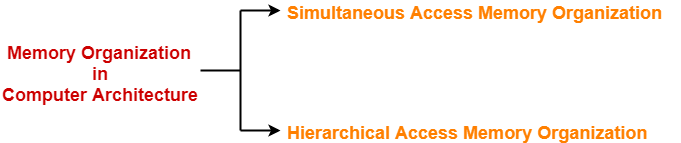1. Simultaneous Access Memory Organization
2. Hierarchical Access Memory Organization

## 1. Simultaneous Access Memory Organization-

In this memory organization,

• All the levels of memory are directly connected to the CPU.
• Whenever CPU requires any word, it starts searching for it in all the levels simultaneously.

## Example-01:

Consider the following simultaneous access memory organization-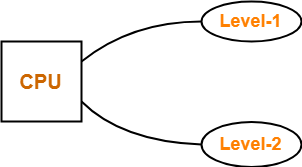Here, two levels of memory are directly connected to the CPU.

Let-

• T1 = Access time of level L1
• S1 = Size of level L1
• C1 = Cost per byte of level L1
• H1 = Hit rate of level L1

Similar are the notations for level L2.

### Average Memory Access Time-

Average time required to access memory per operation

= H1 x T1 + (1 – H1) x H2 x T2

= H1 x T1 + (1 – H1) x 1 x T2

= H1 x T1 + (1 – H1) x T2

### Important Note

In any memory organization,

• The data item being searched will definitely be present in the last level.
• Thus, hit rate for the last level is always 1.

### Average Cost Per Byte-

Average cost per byte of the memory

= { C1 x S1 + C2 x S2 } / { S1 + S2 }

## Example-02:

Consider the following simultaneous access memory organization-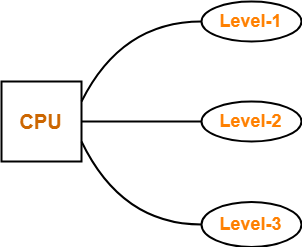Here, three levels of memory are directly connected to the CPU.

Let-

• T1 = Access time of level L1
• S1 = Size of level L1
• C1 = Cost per byte of level L1
• H1 = Hit rate of level L1

Similar are the notations for other two levels.

### Average Memory Access Time-

Average time required to access memory per operation

= H1 x T1 + (1 – H1) x H2 x T2 + (1 – H1) x (1 – H2) x H3 x T3

= H1 x T1 + (1 – H1) x H2 x T2 + (1 – H1) x (1 – H2) x 1 x T3

= H1 x T1 + (1 – H1) x H2 x T2 + (1 – H1) x (1 – H2) x T3

### Average Cost Per Byte-

Average cost per byte of the memory

= { C1 x S1 + C2 x S2 + C3 x S3 } / { S1 + S2 + S3 }

## 2. Hierarchical Access Memory Organization-

In this memory organization, memory levels are organized as-

• Level-1 is directly connected to the CPU.
• Level-2 is directly connected to level-1.
• Level-3 is directly connected to level-2 and so on.

Whenever CPU requires any word,

• It first searches for the word in level-1.
• If the required word is not found in level-1, it searches for the word in level-2.
• If the required word is not found in level-2, it searches for the word in level-3 and so on.

## Example-01:

Consider the following hierarchical access memory organization-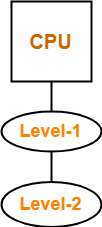Here, two levels of memory are connected to the CPU in a hierarchical fashion.

Let-

• T1 = Access time of level L1
• S1 = Size of level L1
• C1 = Cost per byte of level L1
• H1 = Hit rate of level L1

Similar are the notations for level L2.

### Average Memory Access Time-

Average time required to access memory per operation

= H1 x T1 + (1 – H1) x H2 x (T1 + T2)

= H1 x T1 + (1 – H1) x 1 x (T1 + T2)

= H1 x T1 + (1 – H1) x (T1 + T2)

### Average Cost Per Byte-

Average cost per byte of the memory

= { C1 x S1 + C2 x S2 } / { S1 + S2 }

## Example-02:

Consider the following hierarchical access memory organization-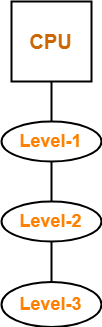Here, three levels of memory are connected to the CPU in a hierarchical fashion.

Let-

• T1 = Access time of level L1
• S1 = Size of level L1
• C1 = Cost per byte of level L1
• H1 = Hit rate of level L1

Similar are the notations for other two levels.

### Average Memory Access Time-

Average time required to access memory per operation

= H1 x T1 + (1 – H1) x H2 x (T1 + T2) + (1 – H1) x (1 – H2) x H3 x (T1 + T2 + T3)

= H1 x T1 + (1 – H1) x H2 x (T1 + T2) + (1 – H1) x (1 – H2) x 1 x (T1 + T2 + T3)

= H1 x T1 + (1 – H1) x H2 x (T1 + T2) + (1 – H1) x (1 – H2) x (T1 + T2 + T3)

### Average Cost Per Byte-

Average cost per byte of the memory

= { C1 x S1 + C2 x S2 + C3 x S3 } / { S1 + S2 + S3 }

## Problem-01:

What is the average memory access time for a machine with a cache hit rate of 80% and cache access time of 5 ns and main memory access time of 100 ns when-

1. Simultaneous access memory organization is used.
2. Hierarchical access memory organization is used.

## Solution-

### Part-01: Simultaneous Access Memory Organization-

The memory organization will be as shown-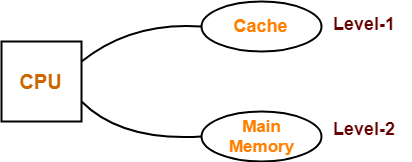Average memory access time

= H1 x T1 + (1 – H1) x H2 x T2

= 0.8 x 5 ns + (1 – 0.8) x 1 x 100 ns

= 4 ns + 0.2 x 100 ns

= 4 ns + 20 ns

= 24 ns

### Part-02: Hierarchical Access Memory Organization-

The memory organization will be as shown-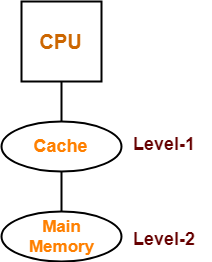Average memory access time

= H1 x T1 + (1 – H1) x H2 x (T1 + T2)

= 0.8 x 5 ns + (1 – 0.8) x 1 x (5 ns + 100 ns)

= 4 ns + 0.2 x 105 ns

= 4 ns + 21 ns

= 25 ns

## Problem-02:

A computer has a cache, main memory and a disk used for virtual memory. An access to the cache takes 10 ns. An access to main memory takes 100 ns. An access to the disk takes 10,000 ns. Suppose the cache hit ratio is 0.9 and the main memory hit ratio is 0.8. The effective access time required to access a referenced word on the system is _______ when-

1. Simultaneous access memory organization is used.
2. Hierarchical access memory organization is used.

## Solution-

### Part-01:Simultaneous Access Memory Organization-

The memory organization will be as shown-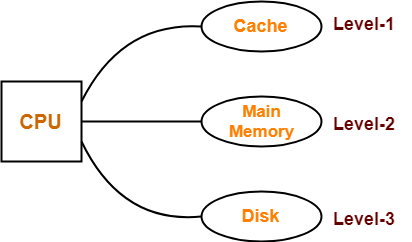Effective memory access time

= H1 x T1 + (1 – H1) x H2 x T2 + (1 – H1) x (1 – H2) x H3 x T3

= 0.9 x 10 ns + (1 – 0.9) x 0.8 x 100 ns + (1 – 0.9) x (1 – 0.8) x 1 x 10000 ns

= 9 ns + 8 ns + 200 ns

= 217 ns

### Part-02: Hierarchical Access Memory Organization-

The memory organization will be as shown-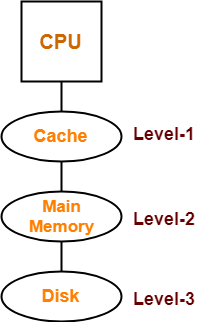Effective memory access time

= H1 x T1 + (1 – H1) x H2 x (T1 + T2) + (1 – H1) x (1 – H2) x H3 x (T1 + T2 + T3)

= 0.9 x 10 ns + (1 – 0.9) x 0.8 x (10 ns + 100 ns) + (1 – 0.9) x (1 – 0.8) x 1 x (10 ns + 100 ns + 10000 ns)

= 9 ns + 8.8 ns + 202.2 ns

= 220 ns

### Important Note

While solving numerical problems

If the kind of memory organization used is not mentioned, assume hierarchical access memory organization.

To gain better understanding about Memory Organization,

Watch this Video Lecture

Next Article- Cache Memory

Get more notes and other study material of Computer Organization and Architecture.

Watch video lectures by visiting our YouTube channel LearnVidFun.

## Memory Hierarchy-

• Memory hierarchy is the hierarchy of memory and storage devices found in a computer system.
• It ranges from the slowest but high capacity auxiliary memory to the fastest but low capacity cache memory.

## Need-

There is a trade-off among the three key characteristics of memory namely-

• Cost
• Capacity
• Access time

Memory hierarchy is employed to balance this trade-off.

## Memory Hierarchy Diagram-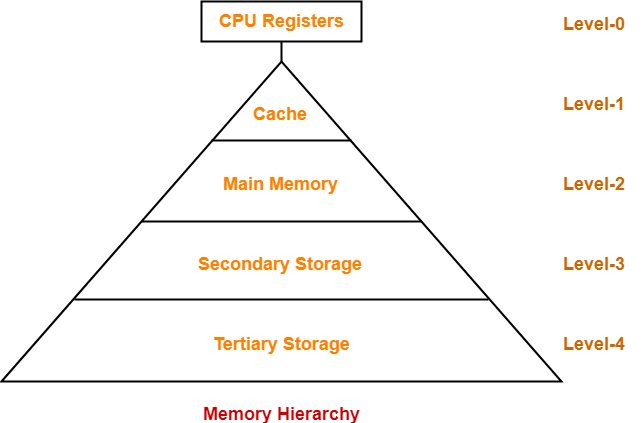### Level-0:

• At level-0, registers are present which are contained inside the CPU.
• Since they are present inside the CPU, they have least access time.
• They are most expensive and therefore smallest in size (in KB).
• Registers are implemented using Flip-Flops.

### Level-1:

• At level-1, Cache Memory is present.
• It stores the segments of program that are frequently accessed by the processor.
• It is expensive and therefore smaller in size (in MB).
• Cache memory is implemented using static RAM.

### Level-2:

• At level-2, main memory is present.
• It can communicate directly with the CPU and with auxiliary memory devices through an I/O processor.
• It is less expensive than cache memory and therefore larger in size (in few GB).
• Main memory is implemented using dynamic RAM.

### Level-3:

• At level-3, secondary storage devices like Magnetic Disk are present.
• They are used as back up storage.
• They are cheaper than main memory and therefore much larger in size (in few TB).

### Level-4:

• At level-4, tertiary storage devices like magnetic tape are present.
• They are used to store removable files.
• They are cheapest and largest in size (1-20 TB).

## Observations-

The following observations can be made when going down in the memory hierarchy-

• Cost / bit decreases
• Frequency of access decreases
• Capacity increases
• Access time increases

## Goals of Memory Hierarchy-

The goals of memory hierarchy are-

• To obtain the highest possible average access speed
• To minimize the total cost of the entire memory system

To gain better understanding about Memory Hierarchy-

Watch this Video Lecture

Next Article- Memory Organization | Simultaneous Vs Hierarchical

Get more notes and other study material of Computer Organization and Architecture.

Watch video lectures by visiting our YouTube channel LearnVidFun.